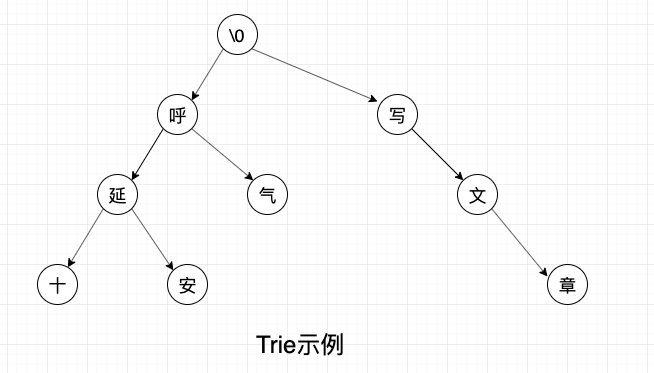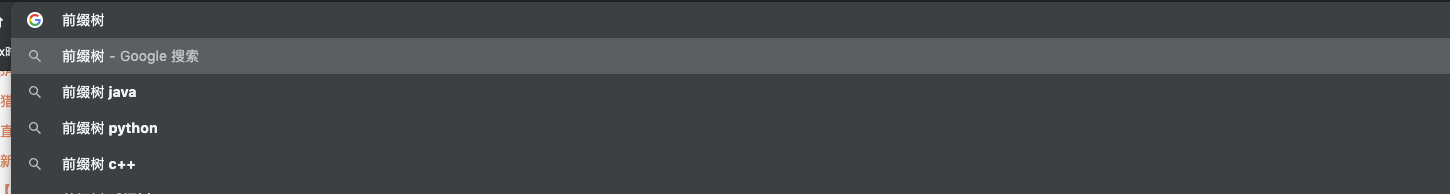# Trie树的基本原理及应用

## 理论知识

### 什么是 Trie 树• 根节点不包含字符，除根节点外的每一个子节点都包含一个字符。
• 从根节点到某一节点，路径上经过的字符连接起来，就是该节点对应的字符串。
• 每个单词的公共前缀作为一个字符节点保存。

Trie 树的每个节点的子节点，是一堆单字符的集合，我们可以很方便的进行对所有字符串进行字典序的排序工作。只需要将字典序先序输出，输出所有子节点时按照字典序遍历即可。所以 Trie 树又叫做字典树。

### Trie 的优劣势

Trie 树的核心思想就是：用空间来换时间，利用字符串的公共前缀来降低查询时间的开销以达到提高效率的目的。

Trie 的强大之处就在于它的时间复杂度，插入和查询的效率很高，都为O(N)，其中 N 是待插入/查询的字符串的长度，而与 Trie 中保存了多少个元素无关。

1. hash 表实现极其简单，且大多数语言都有完善的内部库。使用方便。
2. 大部分时间就 K-V 存储而言，hash 是由于 Trie 树的，尤其是 语言库中经过各方大佬优化的 hash 表。
3. Trie 树要自己实现，且要经过各种逻辑上的测试，保证覆盖率，还要压测等等才能投入使用，成本太高。

### Trie 的应用场景

K-V 存储及检索

trie 树前缀匹配常用于搜索提示。比如各种搜索引擎上的 自动联想后半段功能。## 编码实现

package com.huyan.trie;

import java.util.*;

/**
* Created by pfliu on 2019/12/06.
*/
public class TNode {
/**
* 当前节点字符
*/
private char c;
/**
* 当前 节点对应数字
*/
int count = 0;

private TNode[] children;

private static int hash(char c) {
return c;
}

@Override
public String toString() {
return "TNode{" +
"c=" + c +
", count=" + count +
", children=" + Arrays.toString(children) +
'}';
}

TNode(char c) {
this.c = c;
}

/**
* 将 给定字符  添加到给定列表中。
* @param nodes 给定的 node 列表
* @param c 给定字符
* @return 插入后的节点
*/
private static TNode add(final TNode[] nodes, char c) {
int hash = hash(c);
int mask = nodes.length - 1;

for (int i = hash; i < hash + mask + 1; i++) {
int idx = i & mask;
if (nodes[idx] == null) {
TNode node = new TNode(c);
nodes[idx] = node;
return node;
} else if (nodes[idx].c == c) {
return nodes[idx];
}
}
return null;
}

/**
* 将 当前节点 放入到给定的 节点列表中。
* 用于 resize 的时候转移节点列表
* @param nodes 节点列表
* @param node 给定节点
*/
private static void add(final TNode[] nodes, TNode node) {
int hash = hash(node.c);
int len = nodes.length - 1;

for (int i = hash; i < hash + len + 1; i++) {
int idx = i & len;
if (nodes[idx] == null) {
nodes[idx] = node;
return;
} else if (nodes[idx].c == node.c) {
throw new IllegalStateException("Node not expected for " + node.c);
}
}
}

/**
* 将  给定字符 插入到当前节点的子节点中。
* @param c 给定字符
* @return 插入后的节点
*/
// 初始化子节点列表
if (children == null) {
children = new TNode;
}

// 尝试插入
if (node != null)
return node;

// resize
// 转移节点列表到新的子节点列表中
TNode[] tmp = new TNode[children.length * 2];
for (TNode child : children) {
if (child != null) {
}
}

children = tmp;
}

/**
* 查找当前节点的子节点列表中，char 等于给定字符的节点
* @param c 给定 char
* @return 对应的节点
*/
TNode findChild(char c) {
final TNode[] nodes = children;
if (nodes == null) return null;

int hash = hash(c);
int len = nodes.length - 1;

for (int i = hash; i < hash + len + 1; i++) {
int idx = i & len;
TNode node = nodes[idx];
if (node == null) {
return null;
} else if (node.c == c) {
return node;
}
}
return null;
}
}



package com.huyan.trie;

import java.util.*;

/**
* Created by pfliu on 2019/12/06.
*/
public class Trie {

/**
* 根节点
*/
final private TNode root = new TNode('\0');

/**
* 添加一个词到 Trie
*
* @param word  待添加词
* @param value 对应 value
*/
public void addWord(String word, int value) {
if (word == null || word.length() == 0) return;
TNode node = root;
for (int i = 0; i < word.length(); i++) {
char c = word.charAt(i);
// 当前 char 添加到 trie 中，并拿到当前 char 对应的那个节点
}
node.count = value;
}

/**
* 查找 word 对应的 int 值。
*
* @param word 给定 word
* @return 最后一个节点上存储的 int.
*/
public int get(String word) {
TNode node = root;
for (int i = 0; i < word.length(); i++) {
node = node.findChild(word.charAt(i));
if (node == null) {
return 0;
}
}
return node.count;
}

private int get(char[] buffer, int offset, int length) {
TNode node = root;
for (int i = 0; i < length; i++) {
node = node.findChild(buffer[offset + i]);
if (node == null) {
return 0;
}
}

return node.count;
}

/**
* 从给定字符串的 offset 开始。
* 查找最大匹配的第一个 int 值。
*
* @param str    给定字符串
* @param offset 开始查找的偏移量
* @return 第一个匹配的字符串德最后一个节点的 int 值。
*/
public String maxMatch(String str, int offset) {
TNode node = root;
int lastMatchIdx = offset;

for (int i = offset; i < str.length(); i++) {
char c = str.charAt(i);
node = node.findChild(c);
if (node == null) {
break;
} else if (node.count != 0) {
lastMatchIdx = i;
}
}
return lastMatchIdx == offset ? null : str.substring(offset, lastMatchIdx + 1);
}

/**
* 从给定字符串的 offset <b>反向</b>开始。
* 查找最大匹配的第一个 int 值。
*
* @param str    给定字符串
* @param offset 开始查找的偏移量
* @return 第一个匹配的字符串德最后一个节点的 int 值。
*/
public int maxMatchBack(String str, int offset) {
TNode node = root;
int lastMatchIdx = offset;

for (int i = offset; i >= 0; i--) {
char c = str.charAt(i);
node = node.findChild(c);
if (node == null) {
break;
} else if (node.count != 0) {
lastMatchIdx = i;
}
}
return offset - lastMatchIdx + 1;
}

/**
* 从给定字符串的 offset 开始。检查 length 长度。
* 查找最大匹配的第一个 int 值。
*
* @param buffer 给定字符串
* @param offset 开始查找的偏移量
* @return 第一个匹配的字符串德最后一个节点的 int 值。
*/
public int maxMatch(char[] buffer, int offset, int length) {
TNode node = root;
int lastMatchIdx = offset;

for (int i = offset; i < offset + length; i++) {
char c = buffer[i];
node = node.findChild(c);
if (node == null) {
break;
} else if (node.count != 0) {
lastMatchIdx = i;
}
}
return lastMatchIdx - offset + 1;
}

public static void main(String[] args) {
Trie trie = new Trie();

for (String s : Arrays.asList("呼延", "呼延二十")) {
}

String input = "延十在写文章";

System.out.println(trie.maxMatch(input, 0));

}

}



## 参考文章

https://www.cnblogs.com/huangxincheng/archive/2012/11/25/2788268.html

https://zh.wikipedia.org/wiki/Trie

## 联系我#### ChangeLog

2019-05-19 完成

05-20
09-17743405-014160
04-154756
03-116625
01-0863
10-22473
08-0692
05-172039
05-021万+